Next: Physical Constants Up: Wave Mechanics Previous: Square Potential Barrier

# Exercises

1. Use the standard power law expansions,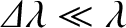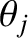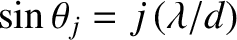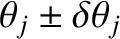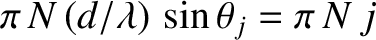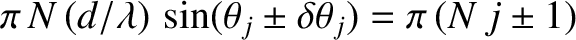which are valid for complex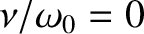, to prove Euler's theorem,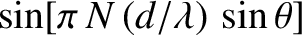where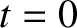is real.
2. Equations (724) and (725) can be combined with Euler's theorem to give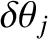where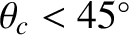is a Dirac delta function. Use this result to prove Fourier's theorem: that is, if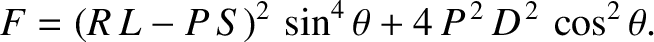then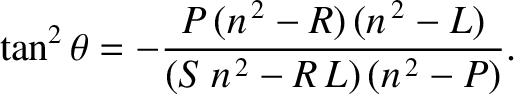3. A He-Ne laser emits radiation of wavelength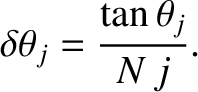. How many photons are emitted per second by a laser with a power of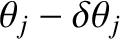? What force does such a laser exert on a body that completely absorbs its radiation?

4. The ionization energy of a hydrogen atom in its ground state is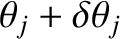. Calculate the frequency (in hertz), wavelength, and wavenumber of the electromagnetic radiation that will just ionize the atom.

5. The maximum energy of photoelectrons from Aluminium is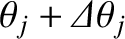for radiation of wavelength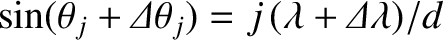, and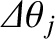for radiation of wavelength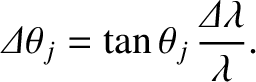. Use this data to calculate Planck's constant (divided by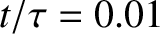) and the work function of Aluminium. [Adapted from Gasiorowicz 1996.]

6. Show that the de Broglie wavelength of an electron accelerated across a potential difference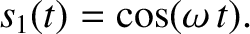is given by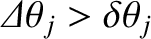whereis measured in volts. [From Pain 1999.]

7. If the atoms in a regular crystal are separated by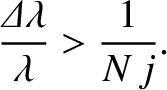demonstrate that an accelerating voltage of about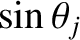would be required to produce a useful electron diffraction pattern from the crystal. [Modified from Pain 1999.]

8. A particle of mass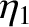has a wavefunction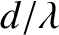whereand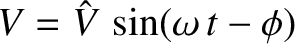are positive real constants. For what potential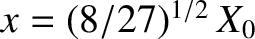does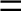satisfy Schrödinger's equation?

9. Show that the wavefunction of a particle of masstrapped in a one-dimensional square potential well of of width, and infinite depth, returns to its original form after a quantum revival time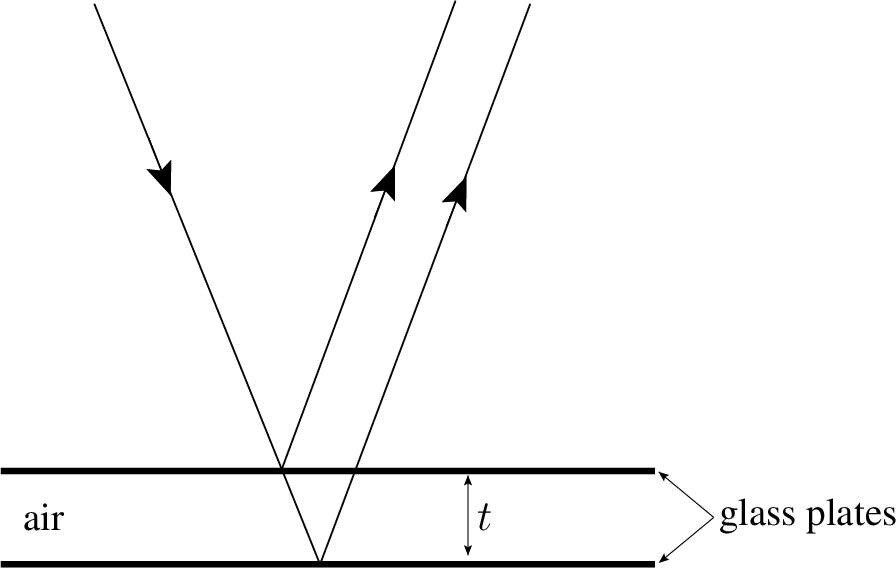.

10. Show that the normalization constant for the stationary wavefunction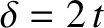describing an electron trapped in a three-dimensional rectangular potential well of dimensions,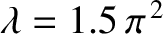,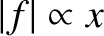, and infinite depth, is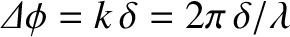. Here,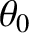,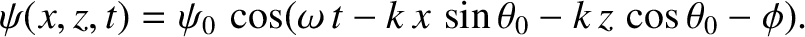, and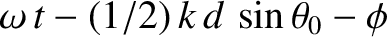are positive integers. [From Pain 1999.]

11. Derive Equation (1193).

12. Consider a particle trapped in the finite potential well whose potential is given by Equation (1179). Demonstrate that for a totally symmetric state the ratio of the probability of finding the particle outside to the probability of finding the particle inside the well is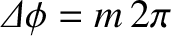where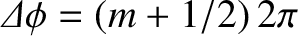, and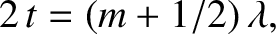. Hence, demonstrate that for a shallow well (i.e.,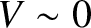)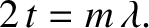, whereas for a deep well (i.e.,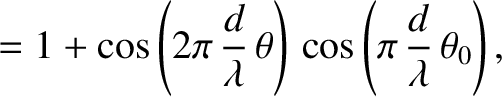)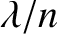(assuming that the particle is in the ground state).

13. Derive expression (1209) from Equations (1205)-(1208).

14. Show that the coefficient of transmission of a particle of massand energy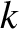, incident on a square potential barrier of height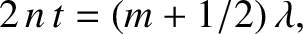, and width, is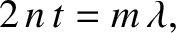where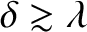and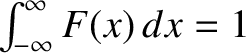. Demonstrate that the coefficient of transmission is unity (i.e., there is no reflection from the barrier) when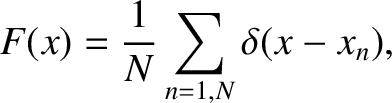, where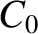is positive integer.

15. The probability of a particle of masspenetrating a distanceinto a classically forbidden region is proportional to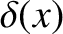, where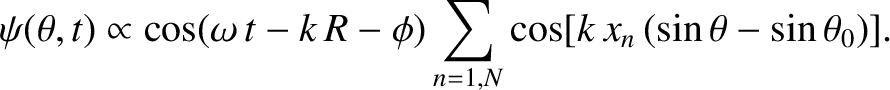If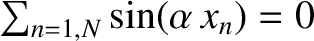and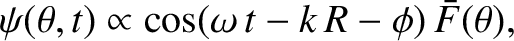show thatis approximately equal to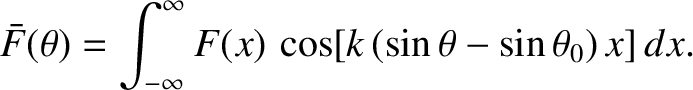for an electron, and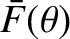for a proton. [Modified from Pain 1999.]Next: Physical Constants Up: Wave Mechanics Previous: Square Potential Barrier
Richard Fitzpatrick 2013-04-08